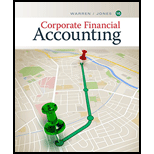# How would the current and quick ratios of a service business compare?### Corporate Financial Accounting

15th Edition
Carl Warren + 1 other
Publisher: Cengage Learning
ISBN: 9781337398169

#### Solutions

Chapter
Section### Corporate Financial Accounting

15th Edition
Carl Warren + 1 other
Publisher: Cengage Learning
ISBN: 9781337398169
Chapter 14, Problem 4DQ
Textbook Problem
23 views

## How would the current and quick ratios of a service business compare?

To determine

Current ratio and acid-test ratio

Current ratio: Current ratio is used to determine the relationship between current assets and current liabilities. The ideal current ratio is 2:1.

Formula:

Current ratio=Current assetsCurrentliabilities

Acid-Test Ratio: This ratio denotes that this ratio is a more rigorous test of solvency than the current ratio. It is determined by dividing quick assets and current liabilities. The acceptable acid-test ratio is 0.90 to 1.00. Use the following formula to determine the acid-test ratio:

Acid Ratio=Quick assetsCurrentliabilities

Quick Assets are those assets that are most liquid. The examples of quick assets include cash and bank balances, marketable securities, and sundry debtors. Use the following formula to determine the Quick assets:

Quick Assets=Current assets (Stock+Prepaid Expenses)

To compare: Current and quick ratios of the service revenue.

### Explanation of Solution

These two ratios are very close as the most service busin...

### Still sussing out bartleby?

Check out a sample textbook solution.

See a sample solution

#### The Solution to Your Study Problems

Bartleby provides explanations to thousands of textbook problems written by our experts, many with advanced degrees!

Get Started

Find more solutions based on key concepts
Explain the following statement: Our tax rates are progressive.

Fundamentals of Financial Management (MindTap Course List)

What are the different forms of compensation?

Foundations of Business (MindTap Course List)

Is backup redundancy inefficient?

Accounting Information Systems

PAYBACK PERIOD Refer to Problem 11-1. What is the projects payback?

Fundamentals of Financial Management, Concise Edition (with Thomson ONE - Business School Edition, 1 term (6 months) Printed Access Card) (MindTap Course List)

Explain why economists usually oppose controls on prices.

Principles of Macroeconomics (MindTap Course List)